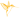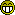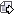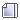###Author Topic: Converting SON coordinates to accurate Lat/Long  (Read 12121 times)

0 Members and 1 Guest are viewing this topic.

####adriank

• Newbie
•• Joined: Jul 2009
• Location: Australia
• Posts: 18
• Unit(s): 997cSI and 998c SI##### Converting SON coordinates to accurate Lat/Long
« on: September 26, 2014, 01:02:28 AM »
Hi

This post is actually a follow on from a previous post http://forums.sideimagingsoft.com/index.php?topic=5623.msg35417 but I've started a new thread as I've made some progress and the question now is slightly different from the previous.

I've been working on extracting the header information from the raw SON files from a 998c SI unit. I have hundreds of recordings so I'm aiming to create a batch extraction of depth and coordinate information. I've been successful at getting out the information from the SON files but the final stumbling block is once again the handling of the coordinates. I now know that the Sonar writes the coordinates in World Mercator metres (EPGS: 3395) thanks to this thread http://gis.stackexchange.com/questions/114765/converting-mercator-meters-without-utm-zone. Unfortunately when I use some spatial transformation libraries (GDAL and ProjNet) the conversion to lat/lon is never accurate enough. I know it is possible to get a very accurate conversion as HumViewer and HBSI Sonar File Converter both produce accurate points with HumViewer the most accurate. Here is a graphic of the various coordinates converted from the same Mercator Meter coordinates 16044360, -4228674.So my question is, what is being done to the raw data to improve its accuracy? According to 'hydrograph' in this thread http://bb.sideimageforums.com/viewtopic.php?t=118&highlight=source+code there probably is some independent axis shift calculation being used. Anyone have an idea what this might be? I don't mind going back to basics and coding my own transformation function but I'm not keen if I'm going to get the same result as other transformation libraries without incorporating the independent shift, if required.

If it's any help to anyone I've attached the header structure from the 998c SI (format borrowed from RGecy http://forums.sideimagingsoft.com/index.php?topic=16.msg67#msg67)Here are a couple of lines data from B000.SON but I don't think the adjustment needed is in it.Any help would be greatly appreciated.

Cheers

####peterv6i##### Re: Converting SON coordinates to accurate Lat/Long
« Reply #1 on: February 08, 2015, 03:14:00 AM »
HumViewer uses this type of conversion:

Code: [Select]
`public class Converter{  private static double R = 6378137.0D;  public static void main(String[] args)  {    Position pos = MMtoEllipsiodDeg(7800892.0D, 1080048.0D);    System.out.println(pos);    System.out.println("Lat/lon =<" + StringUtilities.formatLatitude(pos.latitude) + ", " + StringUtilities.formatLongitude(pos.longitude) + ">");    System.out.println("Lat/lon =<" + StringUtilities.formatDecimalDegreeToDecimalMinutes(Math.abs(pos.latitude), false) + ", " + StringUtilities.formatDecimalDegreeToDecimalMinutes(Math.abs(pos.longitude), false) + ">");    Position p1 = new Position(43.095005D, -89.376367999999999D);    Position p2 = new Position(44.602536999999998D, -92.566222999999994D);    double d = distBetweenPoints(p1, p2);    System.out.println("Dist=<" + d + ">");  }  public static Position MMtoEllipsiodDeg(double Lat_m, double Lon_m)  {    double latitude = MMtoEllipsiodDegLatitude(Lat_m);    double longitude = MMtoEllipsiodDegLongitude(Lon_m);    return new Position(latitude, longitude);  }  public static double MMtoEllipsiodDegLatitude(double Lat_m)  {    if (Math.abs(Lat_m) < 15433199.0D)    {      if (Lat_m != 0.0D) {        return Math.atan(Math.tan(Math.atan(Math.exp(Lat_m / 6378388.0D)) * 2.0D - 1.570796326794897D) * 1.0067642927D) * 57.295779513082302D;      }      return 0.0D;    }    return 0.0D;  }  public static double MMtoEllipsiodDegLongitude(double Lon_m)  {    if (Math.abs(Lon_m) <= 20038300.0D)    {      double d;      if ((d = Lon_m * 57.295779513082302D / 6378388.0D) > 180.0D) {        d = 180.0D;      } else if (d < -180.0D) {        d = -180.0D;      }      return d;    }    return 0.0D;  }  public static double distBetweenPoints(Position pos1, Position pos2)  {    return R * 2.0D * Math.asin(Math.sqrt(Math.pow(Math.sin((Math.toRadians(pos1.latitude) - Math.toRadians(pos2.latitude)) / 2.0D), 2.0D) + Math.cos(Math.toRadians(pos1.latitude)) * Math.cos(Math.toRadians(pos2.latitude)) * Math.pow(Math.sin((Math.toRadians(pos1.longitude) - Math.toRadians(pos2.longitude)) / 2.0D), 2.0D)));  }  public static double calcDist(double length, double depth)  {    return Math.sqrt(Math.pow(length, 2.0D) + Math.pow(depth, 2.0D));  }  public static double slantRateDist(double dist, double depth)  {    double res = Math.sqrt(Math.pow(dist, 2.0D) - Math.pow(depth, 2.0D));    return res;  }  public static Position pointInDistBearing(Position startPos, double dist, double bearing)  {    double lat1 = Math.toRadians(startPos.latitude);    double lon1 = Math.toRadians(startPos.longitude);    double brng = Math.toRadians(bearing);    double lat = Math.asin(Math.sin(lat1) * Math.cos(dist / R) + Math.cos(lat1) * Math.sin(dist / R) * Math.cos(brng));    double lon = lon1 + Math.atan2(Math.sin(brng) * Math.sin(dist / R) * Math.cos(lat1), Math.cos(dist / R) - Math.sin(lat1) * Math.sin(lat));    lon = (lon + 3.141592653589793D) % 6.283185307179586D - 3.141592653589793D;    return new Position(Math.toDegrees(lat), Math.toDegrees(lon));  }  public static double bearingBetweenPoints(Position startPos, Position endPos)  {    double lat1 = Math.toRadians(startPos.latitude);    double lon1 = Math.toRadians(startPos.longitude);    double lat2 = Math.toRadians(endPos.latitude);    double lon2 = Math.toRadians(endPos.longitude);    double bearing = Math.atan2(Math.cos(lat1) * Math.sin(lat2) - Math.sin(lat1) * Math.cos(lat2) * Math.cos(lon2 - lon1), Math.sin(lon2 - lon1) * Math.cos(lat2));    double db = Math.toDegrees(bearing);    return (90.0D - db + 360.0D) % 360.0D;  }  public static double bearingBetweenPointsO(Position startPos, Position endPos)  {    double lat1 = Math.toRadians(startPos.latitude);    double lon1 = Math.toRadians(startPos.longitude);    double lat2 = Math.toRadians(endPos.latitude);    double lon2 = Math.toRadians(endPos.longitude);    double bearing = Math.atan2(Math.sin(lon1 - lon2) * Math.cos(lat2), Math.cos(lat1) * Math.sin(lat2) - Math.sin(lat1) * Math.cos(lat2) * Math.cos(lon1 - lon2));    return Math.toDegrees(bearing % 6.283185307179586D);  }  public static double calcFeetFromMeter(double meter)  {    double factor = 3.28083989501312D;    return factor * meter;  }}`
Code: [Select]
`package HumViewer.util;import HumViewer.ctrl.Configuration;import java.text.DateFormat;import java.text.DecimalFormat;import java.text.SimpleDateFormat;import java.util.Date;import java.util.TimeZone;public class StringUtilities{  protected static final DecimalFormat _floatFormatter1d = new DecimalFormat("0.0");  protected static final DecimalFormat _floatFormatter2d = new DecimalFormat("0.00");  protected static DecimalFormat _0formatter = new DecimalFormat("0");  protected static DecimalFormat _00formatter = new DecimalFormat("00");  protected static DecimalFormat _000formatter = new DecimalFormat("000");  protected static DecimalFormat _00_000formatter = new DecimalFormat("00.000");  protected static DecimalFormat _00_0000formatter = new DecimalFormat("00.0000");  protected static DecimalFormat _0000formatter = new DecimalFormat("0000");  protected static DateFormat _utcDateFormatter = new SimpleDateFormat("dd/MM/yyyy HH:mm:ss");  protected static DateFormat _utcDateFormatterCompact = new SimpleDateFormat("yyyyMMdd HH:mm:ss.S");  protected static DateFormat _iso8601DateTime = new SimpleDateFormat("yyyy-MM-dd'T'HH:mm:ss'Z'");  protected static DateFormat _dateFormatter24 = new SimpleDateFormat("dd/MM/yyyy HH:mm:ss z");  protected static DateFormat _timeFormatter24 = new SimpleDateFormat("HH:mm:ss");  protected static DateFormat _dateFormatter12 = new SimpleDateFormat("dd/MM/yyyy h:mm:ss a z");  protected static DateFormat _timeFormatter12 = new SimpleDateFormat("h:mm:ss a");  protected static DateFormat _timeZoneFormatter = new SimpleDateFormat("z");  protected static DateFormat _dateOnlyFormatter = new SimpleDateFormat("dd/MM/yyyy");  protected static DateFormat _utcDateOnlyFormatter = new SimpleDateFormat("ddMMyy");  protected static DateFormat _utcTimeFormatter = new SimpleDateFormat("HHmmss");  public static final String DEGREE_SIGN = "°"; //new String(new byte[] { -70 });  public static final String DELTA_SIGN = new String(new char[] { 'Δ' });  protected static DecimalFormat _decimalPositionFormatter = null;  protected static int _decimalPositionDigits = -1;  static  {    _utcDateFormatter.setTimeZone(TimeZone.getTimeZone("UTC"));    _iso8601DateTime.setTimeZone(TimeZone.getTimeZone("UTC"));    _utcDateOnlyFormatter.setTimeZone(TimeZone.getTimeZone("UTC"));    _utcTimeFormatter.setTimeZone(TimeZone.getTimeZone("UTC"));  }  public static String formatDateTime(int secsSinceEpoch)  {    String timeUnit = getTimeUnit();    if (timeUnit.equals("12 am/pm")) {      return _dateFormatter12.format(new Date(1000L * secsSinceEpoch));    }    return _dateFormatter24.format(new Date(1000L * secsSinceEpoch));  }  public static String formatDateTimeUTC(int secsSinceEpoch)  {    return _utcDateFormatter.format(new Date(1000L * secsSinceEpoch));  }  public static String formatDateTimeUTC2(long millisSinceEpoch)  {    return _utcDateFormatterCompact.format(new Date(millisSinceEpoch));  }  public static String formatDateTimeIso8601(int secsSinceEpoch)  {    return _iso8601DateTime.format(new Date(1000L * secsSinceEpoch));  }  public static String formatTime(int secsSinceEpoch)  {    String timeUnit = getTimeUnit();    if (timeUnit.equals("12 am/pm")) {      return _timeFormatter12.format(new Date(1000L * secsSinceEpoch));    }    return _timeFormatter24.format(new Date(1000L * secsSinceEpoch));  }  public static String formatDateOnlyUTC(long millisSinceEpoch)  {    return _utcDateOnlyFormatter.format(new Date(millisSinceEpoch));  }  public static String formatTimeUTC(long millisSinceEpoch)  {    return _utcTimeFormatter.format(new Date(millisSinceEpoch));  }  public static String getTimeUnit()  {    return Configuration.getProgramConf().getConfigValue("Units", "Time", "24");  }  public static String formatDate(int secsSinceEpoch)  {    return _dateOnlyFormatter.format(new Date(1000L * secsSinceEpoch));  }  public static String getTimeZone(int secsSinceEpoch)  {    return _timeZoneFormatter.format(new Date(1000L * secsSinceEpoch));  }  public static String formatDepth(double depth, int numDigits)  {    DecimalFormat formatter;    if (numDigits == 0)    {      formatter = _0formatter;    }    else    {      if (numDigits == 1) {        formatter = _floatFormatter1d;      } else {        formatter = _floatFormatter2d;      }    }    if (getDepthUnit().equals("m")) {      return formatter.format(depth);    }    return formatter.format(3.280839920043945D * depth);  }  public static String formatDepth10cm(int depth_10cm, int numDigits)  {    return formatDepth(depth_10cm / 10.0D, numDigits);  }  public static String getDepthUnit()  {    return Configuration.getProgramConf().getConfigValue("Units", "Depth", "m");  }  public static String formatSpeed(int speedx10)  {    String speedUnit = getSpeedUnit();    if (speedUnit.equals("m/s")) {      return _floatFormatter1d.format(speedx10 / 10.0D);    }    if (speedUnit.equals("km/h")) {      return _floatFormatter1d.format(0.3600000143051148D * speedx10);    }    if (speedUnit.equals("mph")) {      return _floatFormatter1d.format(0.2237000018358231D * speedx10);    }    if (speedUnit.equals("kts")) {      return _floatFormatter1d.format(0.1943839937448502D * speedx10);    }    return _floatFormatter1d.format(speedx10 / 10.0D);  }  public static String formatSpeedKts(int speedx10)  {    return _floatFormatter1d.format(0.1943839937448502D * speedx10);  }  public static String formatHeading(int headingx10)  {    return _floatFormatter1d.format(headingx10 / 10.0D);  }  public static String getSpeedUnit()  {    return Configuration.getProgramConf().getConfigValue("Units", "Speed", "m/s");  }  public static String formatSeconds(int seconds)  {    int hours = seconds / 3600;    int min = (int)(seconds / 60.0F % 60.0F);    int sec = seconds % 60;    return _00formatter.format(hours) + ":" + _00formatter.format(min) + ":" + _00formatter.format(sec);  }  public static String formatMillisSeconds(int millis)  {    int seconds = millis / 1000;    int hours = seconds / 3600;    int min = (int)(seconds / 60.0F % 60.0F);    int sec = seconds % 60;    int mil = millis % 1000;    return _00formatter.format(hours) + ":" + _00formatter.format(min) + ":" + _00formatter.format(sec) + "." + _000formatter.format(mil);  }  public static String getPositionUnit()  {    return Configuration.getProgramConf().getConfigValue("Units", "Position", "DDDº MM.MMM'(N/S/W/E)");  }  public static String formatDecimalDegreeToDDMMSS_S(double deg)  {    int degree = (int)deg;    double decMin = 60.0D * (deg - degree);    int minutes = (int)decMin;    double decSecs = 60.0D * (decMin - minutes);    int seconds = (int)decSecs;    int millis = (int)(1000.0D * (decSecs - seconds));    return degree + DEGREE_SIGN + _00formatter.format(minutes) + "'" + _00formatter.format(seconds) + "." + _000formatter.format(millis) + "\"";  }  public static String formatDecimalDegreeToDecimalMinutes(double deg, boolean degreeSign)  {    int degree = (int)deg;    double decMin = 60.0D * (deg - degree);    return degree + (degreeSign ? DEGREE_SIGN : "") + " " + _00_000formatter.format(decMin);  }  public static String formatDecimalDegreeToNMEA(double deg)  {    int degree = (int)deg;    double decMin = 60.0D * (deg - degree);    return _000formatter.format(degree) + _00_0000formatter.format(decMin);  }  public static String formatDecimalDegreeToString(double deg)  {    if (_decimalPositionDigits != Configuration.getProgramConf().getIntConfigValue("Units", "PositionNumDigits", 6))    {      _decimalPositionDigits = Configuration.getProgramConf().getIntConfigValue("Units", "PositionNumDigits", 6);      String dFormat = "0.";      for (int i = 0; i < _decimalPositionDigits; i++) {        dFormat = dFormat + "0";      }      _decimalPositionFormatter = new DecimalFormat(dFormat);    }    return _decimalPositionFormatter.format(deg);  }  public static String formatLatitude(double lat)  {    boolean north = lat >= 0.0D;    if (getPositionUnit().equals("DDDº MM.MMM'(N/S/W/E)")) {      return formatDecimalDegreeToDecimalMinutes(Math.abs(lat), true) + (north ? "N" : "S");    }    if (getPositionUnit().equals("DDD MM.MMM'(N/S/W/E)")) {      return formatDecimalDegreeToDecimalMinutes(Math.abs(lat), false) + (north ? "N" : "S");    }    if (getPositionUnit().equals("(N/S/W/E)DDD MM.MMM'")) {      return (north ? "N" : "S") + formatDecimalDegreeToDecimalMinutes(Math.abs(lat), false);    }    return formatDecimalDegreeToString(lat);  }  public static String formatLongitude(double lon)  {    boolean east = lon >= 0.0D;    if (getPositionUnit().equals("DDDº MM.MMM'(N/S/W/E)")) {      return formatDecimalDegreeToDecimalMinutes(Math.abs(lon), true) + (east ? "E" : "W");    }    if (getPositionUnit().equals("DDD MM.MMM'(N/S/W/E)")) {      return formatDecimalDegreeToDecimalMinutes(Math.abs(lon), false) + (east ? "E" : "W");    }    if (getPositionUnit().equals("(N/S/W/E)DDD MM.MMM'")) {      return (east ? "E" : "W") + formatDecimalDegreeToDecimalMinutes(Math.abs(lon), false);    }    return formatDecimalDegreeToString(lon);  }}`

####adriank

• Newbie
•• Joined: Jul 2009
• Location: Australia
• Posts: 18
• Unit(s): 997cSI and 998c SI##### Re: Converting SON coordinates to accurate Lat/Long
« Reply #2 on: February 09, 2015, 05:43:01 PM »
peterv6i you're a legend.The code worked spot on. This will save me a lot of time.

Thank you very much for that.

Cheers

####BobGinCO##### Re: Converting SON coordinates to accurate Lat/Long
« Reply #3 on: September 02, 2015, 04:03:12 PM »
OK, I get all this... but what does the class definition for Position look like?
Live at 9,000 feet elevation.
Boat at 7,500 feet elevation...
Where my Merc 115 generates about 91.5 HP...

####peterv6i##### Re: Converting SON coordinates to accurate Lat/Long
« Reply #4 on: September 03, 2015, 02:28:20 AM »
Code: [Select]
`package HumViewer.Model;public class Position{  public double latitude;  public double longitude;    public Position(double latitude, double longitude)  {    this.latitude = latitude;    this.longitude = longitude;  }    public String toString()  {    return "Position =<" + this.latitude + ", " + this.longitude + ">";  }}`

####BobGinCO##### Re: Converting SON coordinates to accurate Lat/Long
« Reply #5 on: September 03, 2015, 04:52:49 PM »
Thanks!  That's pretty simple!

Of course, that class isn't needed for the conversion of the mercator meters to lat and long, but it is needed for some of the other conversion class methods.  I see that they depend heavily on java's math class, and i am not using that, but rather c# .NET math class, so I did have to write my own toradians and todegrees methods, but those are simple.

It's surprising how many duplicate pings you get out of the B001.SON files...well, not really, but i wrote my .SON to CSV converter to skip the duplicates, in some cases reducing the data set by about 20%.
Live at 9,000 feet elevation.
Boat at 7,500 feet elevation...
Where my Merc 115 generates about 91.5 HP...

####Caledonia

• Newbie
•• Joined: Jan 2014
• Posts: 1##### Re: Converting SON coordinates to accurate Lat/Long
« Reply #6 on: September 18, 2015, 03:27:34 AM »
Is there a program that will become public? I think there is a demand for this particular program.
Very well done

Greetings Martin

####BobGinCO##### Re: Converting SON coordinates to accurate Lat/Long
« Reply #7 on: September 18, 2015, 11:20:56 AM »
Well, I've written a simple c#.NET console app that reads the .SON files and converts them to .CSV files - it processes about 75 recordings with about 3 million pings in 130 MB to 75 .CSV files in less than a minute.  I wouldn't say it's ready to be released for general use yet, but I'm about to start working in earnest on it in the next few weeks.  One of the challenges is that the actual create date/time for the recording is in the .DAT file, not the .SON file, so I have to add that to the processing, because my lake can vary in surface elevation as much as 60 feet (20 M) throughout the year, so if I want to establish true bottom elevations, I have to compensate for water level at the time the recording was made.
Live at 9,000 feet elevation.
Boat at 7,500 feet elevation...
Where my Merc 115 generates about 91.5 HP...

####gpscharts

• Newbie
•• Joined: Oct 2013
• Location: Ventnor New Jersey USA
• Posts: 1
• Unit(s): 998
• Accessories: towfish##### Re: Converting SON coordinates to accurate Lat/Long
« Reply #8 on: April 02, 2019, 06:47:59 PM »
Will your solution work on an ONIX datafile?

### Related Topics

Subject / Started by Replies Last post1 Replies
8200 ViewsMarch 17, 2011, 01:51:12 PM
by xSilmarilSx7 Replies
17508 ViewsAugust 15, 2011, 04:48:37 AM
by Rüdiger6 Replies
17150 ViewsJanuary 22, 2012, 09:18:22 PM
by Fish Pirate6 Replies
12402 ViewsOctober 29, 2012, 12:53:03 PM
by Humminbird_Greg13 Replies
15613 ViewsAugust 30, 2013, 09:26:36 AM
by SonarGene1 Replies
6783 ViewsJanuary 15, 2014, 09:09:43 PM
by LittleGazoo4 Replies
16953 ViewsJune 02, 2014, 03:40:55 AM
by sfw19606 Replies
3110 ViewsJuly 13, 2021, 10:06:38 PM
by rnvinc

anything